# Biot-Savart Law

## Magnetics of Class 12

This law tells us about the magnetic field (magnitude and direction) produced by moving charges. The Biot-Savart law is a mathematical description of the magnetic field d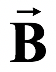that arises from a current I flowing along an infinitesimal path element d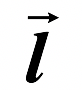called the current element.

The four properties of the magnetic field are as follows.1. The magnetic field grows weaker as we move farther from its source. In particular, the magnitude of the magnetic field dis inversely proportional to the square of the distance from the current element Id.

2. The larger the electric current, the larger is the magnetic field. In particular, the magnitude of the magnetic field dis proportional to the current I.

3. The magnitude of the magnetic field dis proportional to sin θ, where θ is the angle between the direction of current element Idand vector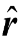that points from the current element to the point in space where dB is evaluated.

4. The direction of the-is not radially away from its source as the gravitational field and the electric field are from their sources. In fact, the direction of dis perpendicular to both Idl and the vector.

These properties of the magnetic field can be written mathematically as

d=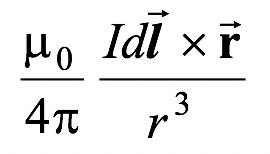or d=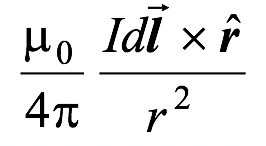(3.1)

 whereis a unit vector that points from the current element to the point in space where dis to be evaluated. The constant μo is called the permeability of free space or the permeability constant. Its value is defined to be μo = 4π × 10−7 T m/A The direction of the magnetic field element dB as given by Biot Savart law is shown in the figure (3.1). The direction ofcan be obtained by using the magnitude of the cross product in equation (3.1).dB =(3.2)

The direction ofcan be obtained by applying right hand on Id×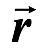.

For a conducting path of finite length, the total magnetic field B at any given point in space is computed by summing the contributions from each small element of the current along its total path. It can be done by integrating equation (3.2) along the current path made up of dl vectors. In practice one comes across two different cases, each requiring different strategy.

Case 1

The dcontributions from each Idall point in the same direction (or at worst in opposite directions). In this situation one can integrate immediately using the magnitude expression in equation (3.2).

Case 2

The dcontributions from I dsources located on different parts of conductor point in different directions. In this case, before integration one must resolve the dcontributions into components.

Case 1 occurs whenever the lines of current and the observation point lie in the same plane.

Example : 3.1

Find the field strength at a distance R from an infinite straight wire that carries a current I.

Solution

Fig. (3.3) shows the infinitesimal contribution to the field, dfrom an arbitrary current element. In order to integrate contributions of all the elements, we use the angle α, measure from the perpendicular, as the variable. From the diagram we see that

|d×| = dl sin θ

= dl sin (π/2 + α)

= dl cos α

Since l = R tan α, we have

dl = R sec2 α dα.

Furthermore,

r = R sec α

 On substituting these expression into equation (3.1) and integrating we find B ==(sin α1 + sin α2) (3.3) Note that angles on opposite sides of the perpendicular are assigned opposite signs. This ensures that the contributions from either side of the wire add together rather than subtract. For an infinite wire, the limits of integration are -π/2 to +π/2. Thus B =(3.4)Example : 3.2

A circular loop of radius a carries a current I. Find the magnetic field along the axis of the loop at a distance x from the centre.

Solution

 Fig. (3.4) shows the infinitesimal contribution to the field dfrom an arbitrary current element Idl. (use the right-hand rule to confirm the direction of the field). The field increment dhas components both along and perpendicular to the axis. However, if we consider the contributions of current element that are diametrically opposite, we see that their components normal to the axis will cancel. Since dandare perpendicular, it follows that |d×| = rdlTherefore, the component of dB along the axis is

dB|| = dB sin α =The total field strength is given by the integral of this expression over all elements. Since the only variable is l, the integral reduces to a sum of length elements.

B|| =Since ∫ dI = 2πa and r2 = a2 + x2, we may express the result as

B|| =(i) (3.5)

Note that this expression applies only to points on the axis of the loop.

For points far from the loop, that is, when x >> a, (i) becomes,

B|| =(3.6)

where pm = I(πa2).

Example: 3.3

A solenoid of length l and radius a has N turns of wire and carries a current I. Find the field strength at a point along the axis.

Solution

Since the solenoid is a series of closely packed loops. We may use equation (3.5) for the field on the axis of a current carrying loop. The solenoid is divided into current loops of width dy, as in figure (3.5a), each of which contains ndy turns, where n = N/l is the number of turns per unit length. The current within such a loop is (n dy)I. From the diagram we see that

y = a tan θ

from which dy = a sec2θ dθ

Thus, I in equation (3.5) is replaced by

nIdy = nIa sec2θ dθ

After substituting for I and y in equation (3.5) and simplifying, we find

dB =μo nI cos θ dθ

#### For a finite solenoid, the total field strength is

B = 1/2 μonI ∫θ2θ1 cos θ dθ = 1/2μonI (sin θ2 − sin θ1) (3.7)Fig.(3.5) (a) To calculate the field strength along the axis of a solenoid, the solenoid is divided into infinitesimal loops.

(b) The solenoid subtends an angle θ1 and θ2 at the point where we have to obtain the magnetic field.

If the solenoid is infinite long, θ1 = −90° and θ2 = +90°, therefore, the field at the axis of the solenoid is

B = μonI (3.8)

Example : 3.4

(a) What is the magnetic field produced by a point charge q moving at velocity v ?

(b) What is the force between two equal charges moving parallel to each other with the same velocity ?

Solution

(a) The Biot-Savart law refers the magnetic field produced by a current element, Id.

Since I = dq/dt we rewrite

Id= dq/dt d= dq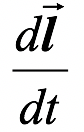= (dq)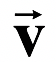whereis the velocity of the charge q. From equation (3.1), we infer that the field due to a single charge q moving at velocity v is=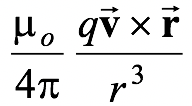(3.9) The magnetic field lines are circular, as shown in figure, (3.6 a). (b) In Fig.(3.6 b), the repulsive force between the charges is FE =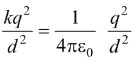The magnitude of the magnetic force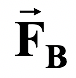= q×exerted by one charge on the other is FB = (qv)This force is attractive. The net forced between the charge is F = FE – FB =Since c2 =, therefore, F =The net force on each of the particles moving with the same velocity is less than that when they are at rest.

Table 3.1 Magnetic field due to different current systems

 1. A straight wire of finite lengthB = μoI/4πR(sinα1 + sinα2) 2. An infinitely long straight wireB = μoI/2πR 3. An arc of a circleB =4. On the axis of a RingB⊥ = 0 B|| =5. At the centre of a Ring B = μoI /2R 6. On the axis of a solenoidB = μonI = μoN/l I where N is the total number of turns and n is the number of turns per unit length. Assuming that radius of the loop is very small compared to its length.